Test: Arithmetic And Geometric Progressions - 1

# Test: Arithmetic And Geometric Progressions - 1

Test Description

## 40 Questions MCQ Test Business Mathematics and Logical Reasoning & Statistics | Test: Arithmetic And Geometric Progressions - 1

Test: Arithmetic And Geometric Progressions - 1 for CA Foundation 2023 is part of Business Mathematics and Logical Reasoning & Statistics preparation. The Test: Arithmetic And Geometric Progressions - 1 questions and answers have been prepared according to the CA Foundation exam syllabus.The Test: Arithmetic And Geometric Progressions - 1 MCQs are made for CA Foundation 2023 Exam. Find important definitions, questions, notes, meanings, examples, exercises, MCQs and online tests for Test: Arithmetic And Geometric Progressions - 1 below.
Solutions of Test: Arithmetic And Geometric Progressions - 1 questions in English are available as part of our Business Mathematics and Logical Reasoning & Statistics for CA Foundation & Test: Arithmetic And Geometric Progressions - 1 solutions in Hindi for Business Mathematics and Logical Reasoning & Statistics course. Download more important topics, notes, lectures and mock test series for CA Foundation Exam by signing up for free. Attempt Test: Arithmetic And Geometric Progressions - 1 | 40 questions in 40 minutes | Mock test for CA Foundation preparation | Free important questions MCQ to study Business Mathematics and Logical Reasoning & Statistics for CA Foundation Exam | Download free PDF with solutions
 1 Crore+ students have signed up on EduRev. Have you?
Test: Arithmetic And Geometric Progressions - 1 - Question 1

### Choose the most appropriate option ( a ), ( b ) , ( c ) or (d) The nth element of the sequence 1, 3, 5, 7,….…..Is

Detailed Solution for Test: Arithmetic And Geometric Progressions - 1 - Question 1

Let a be the first term and d be the common difference.
Given series is in AP.

a = 1
d = 3 - 1 = 2

We know that nth term of an Ap an = a + (n - 1) * d

= 1 + (n - 1) * 2
= 1 + 2n - 2
= 2n - 1.

Test: Arithmetic And Geometric Progressions - 1 - Question 2

### The nth element of the sequence –1, 2, –4, 8 ….. is

Detailed Solution for Test: Arithmetic And Geometric Progressions - 1 - Question 2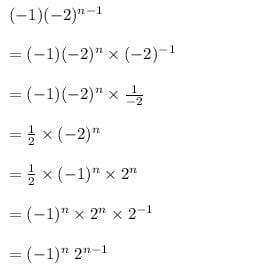Test: Arithmetic And Geometric Progressions - 1 - Question 3

### The arithmetic mean between a and 10 is 30, the value of ‘a’ should be

Test: Arithmetic And Geometric Progressions - 1 - Question 4

–5, 25, –125 , 625, ….. can be written as

Test: Arithmetic And Geometric Progressions - 1 - Question 5

The first three terms of sequence when nth term tn is n2 – 2n are

Test: Arithmetic And Geometric Progressions - 1 - Question 6

Which term of the progression –1, –3, –5, …. Is –39

Test: Arithmetic And Geometric Progressions - 1 - Question 7

The value of x such that 8x + 4, 6x – 2, 2x + 7 will form an AP is

Test: Arithmetic And Geometric Progressions - 1 - Question 8

The mth term of an A. P. is n and nth term is m. The r th term of it is

Test: Arithmetic And Geometric Progressions - 1 - Question 9

An infinite GP has first term x and sum 5, then x belongs to

Detailed Solution for Test: Arithmetic And Geometric Progressions - 1 - Question 9

We know that, the sum of infinite terms of GP is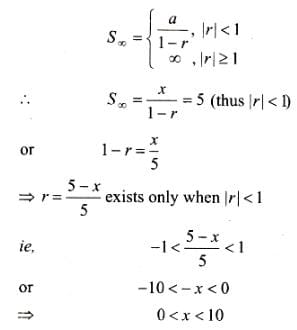Test: Arithmetic And Geometric Progressions - 1 - Question 10

The nth term of the series whose sum to n terms is 5n2 + 2n is

Detailed Solution for Test: Arithmetic And Geometric Progressions - 1 - Question 10

It is given that the sum of n terms is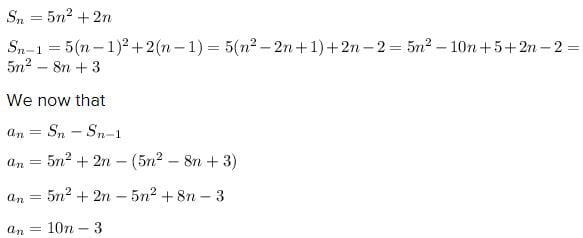Test: Arithmetic And Geometric Progressions - 1 - Question 11

The 20th term of the progression 1, 4, 7, 10.................is

Test: Arithmetic And Geometric Progressions - 1 - Question 12

The last term of the series 5, 7, 9,….. to 21 terms is

Test: Arithmetic And Geometric Progressions - 1 - Question 13

The last term of the A.P. 0.6, 1.2, 1.8,… to 13 terms is

Test: Arithmetic And Geometric Progressions - 1 - Question 14

The sum of the series 9, 5, 1,…. to 100 terms is

Test: Arithmetic And Geometric Progressions - 1 - Question 15

The two arithmetic means between –6 and 14 is

Detailed Solution for Test: Arithmetic And Geometric Progressions - 1 - Question 15

Let the terms be – 6, a, b 14

a = – 6

T4 = a + 3d = 14

– 6 + 3d = 14

3d = 20

d = 20/3

a = – 6 + 20/3 = 2/3

b = 2/3 + 20/3 = 22/3

Test: Arithmetic And Geometric Progressions - 1 - Question 16

The sum of three integers in AP is 15 and their product is 80. The integers are

Detailed Solution for Test: Arithmetic And Geometric Progressions - 1 - Question 16

let a - d , a , a + d are three terms of an AP

according to the problem given,

sum of the terms = 15

a - d + a + a + d = 15

3a = 15

a = 15 / 3

a = 5

product = 80

( a - d ) a ( a + d ) = 80

( a² - d² ) a = 80

( 5² - d² ) 5 = 80

25 - d² = 80 /5

25 - d² = 16

- d² = - 9

d² = 3²

d = ± 3

Therefore,

a = 5 , d = ±3

required 3 terms are

a - d = 5 - 3 = 2

a = 5

a+ d = 5 + 3 = 8

( 2 , 5 , 8 ) or ( 8 , 5 , 2 )

Test: Arithmetic And Geometric Progressions - 1 - Question 17

The sum of n terms of an AP is 3n2 + 5n. A.P. is

Detailed Solution for Test: Arithmetic And Geometric Progressions - 1 - Question 17

The sum of n terms of an A.P. = 3n2+5n

then sum of n-1 terms= 3(n-1)2+5(n-1)

So nth term of A.P will be Tn=3n2+5n- 3(n-1)2-5(n-1)= 3n2+5n-3n2-3+6n-5n+5=6n+2

in this way by putinng values of n= 1,2,3,4,5,6,7,_______n-1, n

we will get the AP as 8,14,20,26,32,38,44,____

Test: Arithmetic And Geometric Progressions - 1 - Question 18

The number of numbers between 74 and 25556 divisible by 5 is

Test: Arithmetic And Geometric Progressions - 1 - Question 19

The pth term of an AP is (3p – 1)/6. The sum of the first n terms of the AP is

Test: Arithmetic And Geometric Progressions - 1 - Question 20

The arithmetic mean between 33 and 77 is

Test: Arithmetic And Geometric Progressions - 1 - Question 21

The 4 arithmetic means between –2 and 23 are

Detailed Solution for Test: Arithmetic And Geometric Progressions - 1 - Question 21

a=-2 n=6

given : t6= 23

23= a+5d

23= -2+5d

d= 25/5

d = 5

t2= a+d= -2+5=3

t3= 8

t4= 13

t5= 18

Test: Arithmetic And Geometric Progressions - 1 - Question 22

The first term of an A.P is 14 and the sums of the first five terms and the first ten terms are equal is magnitude but opposite in sign. The 3rd term of the AP is

Test: Arithmetic And Geometric Progressions - 1 - Question 23

The sum of a certain number of terms of an AP series –8, –6, –4, …… is 52. The number of terms is

Test: Arithmetic And Geometric Progressions - 1 - Question 24

The 1st and the last term of an AP are –4 and 146. The sum of the terms is 7171. The number of terms is

Test: Arithmetic And Geometric Progressions - 1 - Question 25

The sum of the series 3 ½ + 7 + 10 ½ + 14 + …. To 17 terms is

Test: Arithmetic And Geometric Progressions - 1 - Question 26

The 7th term of the series 6, 12, 24,……is

Test: Arithmetic And Geometric Progressions - 1 - Question 27

t8 of the series 6, 12, 24,…is

Test: Arithmetic And Geometric Progressions - 1 - Question 28

t12 of the series –128, 64, –32, ….is

Test: Arithmetic And Geometric Progressions - 1 - Question 29

The 4th term of the series 0.04, 0.2, 1, … is

Test: Arithmetic And Geometric Progressions - 1 - Question 30

The last term of the series 1, 2, 4,…. to 10 terms is

Test: Arithmetic And Geometric Progressions - 1 - Question 31

The last term of the series 1, –3, 9, –27 up to 7 terms is

Test: Arithmetic And Geometric Progressions - 1 - Question 32

The last term of the series x2, x, 1, …. to 31 terms is

Test: Arithmetic And Geometric Progressions - 1 - Question 33

The sum of the series –2, 6, –18, …. To 7 terms is

Test: Arithmetic And Geometric Progressions - 1 - Question 34

The sum of the series 24, 3, 8, 1, 2, 7,… to 8 terms is

Detailed Solution for Test: Arithmetic And Geometric Progressions - 1 - Question 34

Sum of series 24,3,8,1,2,7..to 8th term is:
Take the 1st and 2nd terms, 24,3, put them together, 243 = 3^5.
Take the 3rd and 4th terms, 8,1, put them together, 81 = 3^4.
Take the 5th and 6th terms, 2,7, put them together, 27 = 3^3.
Take the 7th and 8th terms, 0,9, put them together, 09 = 3^2.
Sum of 8 terms = 24+3+8+1+2+7+0+9 = 54

Test: Arithmetic And Geometric Progressions - 1 - Question 35

The sum of the series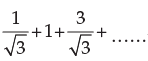to 18 terms is

Test: Arithmetic And Geometric Progressions - 1 - Question 36

The second term of a G P is 24 and the fifth term is 81. The series is

Test: Arithmetic And Geometric Progressions - 1 - Question 37

The sum of 3 numbers of a G P is 39 and their product is 729. The numbers are

Test: Arithmetic And Geometric Progressions - 1 - Question 38

In a G. P, the product of the first three terms 27/8. The middle term is

Test: Arithmetic And Geometric Progressions - 1 - Question 39

If you save 1 paise today, 2 paise the next day 4 paise the succeeding day and so on, then your total savings in two weeks will be

Detailed Solution for Test: Arithmetic And Geometric Progressions - 1 - Question 39

If we save 1 paise today,2 paise the next day,4 pause the succeeding day and so on then you total savings in two weeks the sequence should be Geometric progression .

1, ,2,4, 8, 16, .....,14 terms

First term (a) = 1,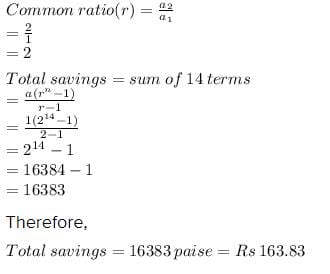Test: Arithmetic And Geometric Progressions - 1 - Question 40

Sum of n terms of the series 4 + 44 + 444 + … is

Detailed Solution for Test: Arithmetic And Geometric Progressions - 1 - Question 40

S=4+44+444+-------n terms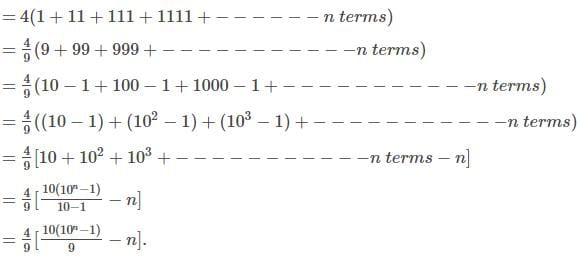## Business Mathematics and Logical Reasoning & Statistics

131 videos|149 docs|87 tests
 Use Code STAYHOME200 and get INR 200 additional OFF Use Coupon Code
Information about Test: Arithmetic And Geometric Progressions - 1 Page
In this test you can find the Exam questions for Test: Arithmetic And Geometric Progressions - 1 solved & explained in the simplest way possible. Besides giving Questions and answers for Test: Arithmetic And Geometric Progressions - 1, EduRev gives you an ample number of Online tests for practice

## Business Mathematics and Logical Reasoning & Statistics

131 videos|149 docs|87 tests